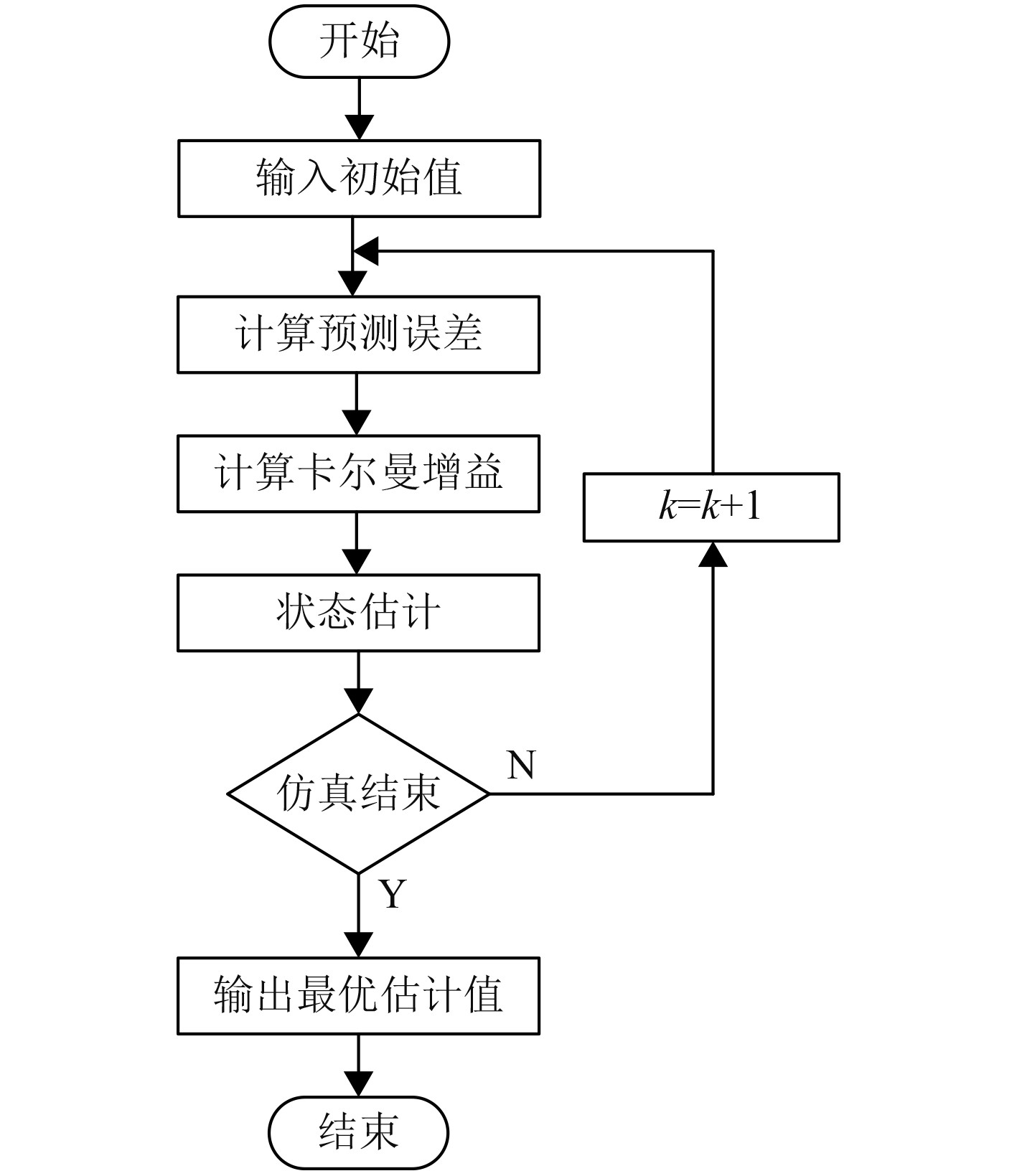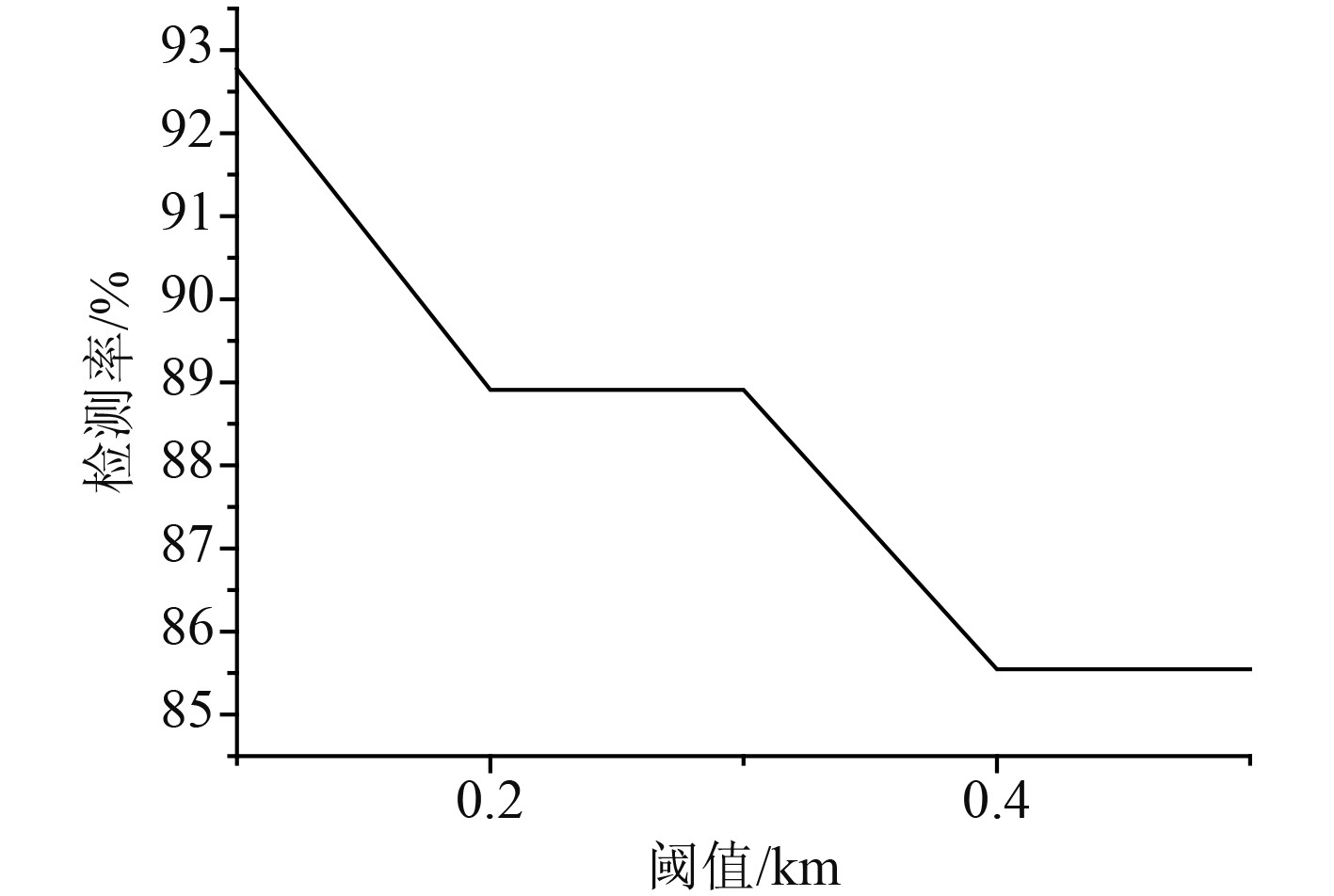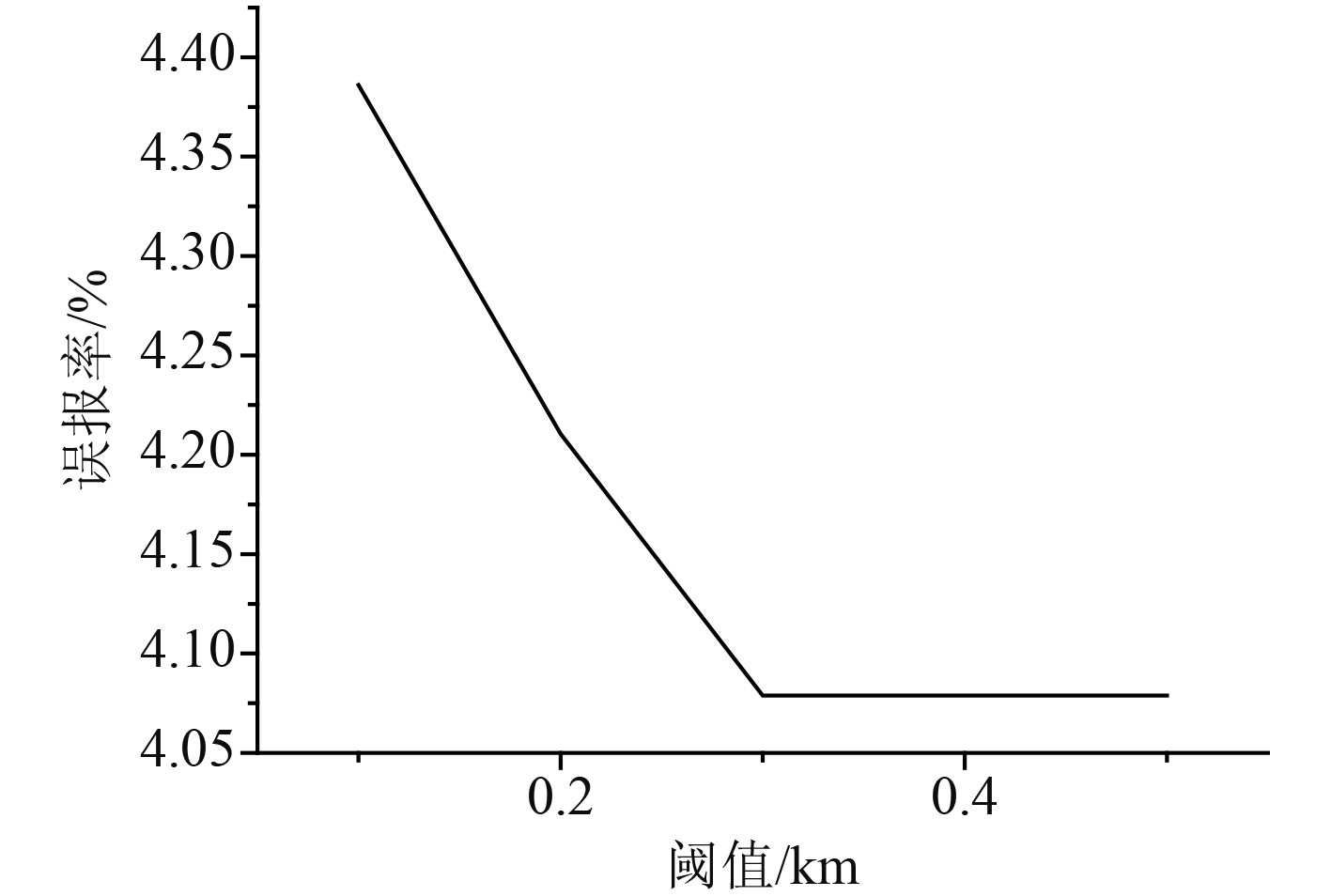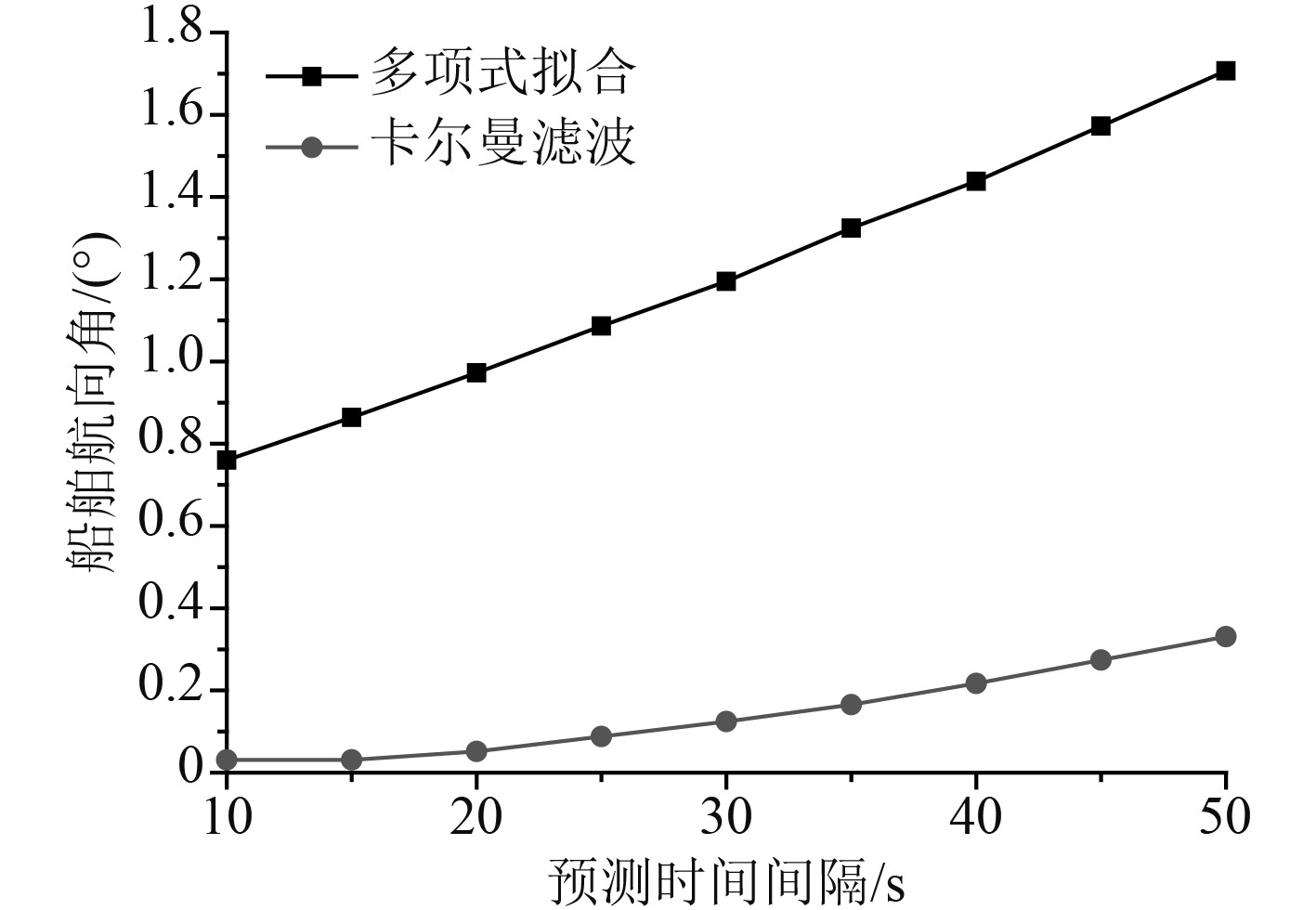﻿ 基于卡尔曼滤波预测的轨迹异常检测方法
 舰船科学技术2022, Vol. 44Issue (14): 73-76    DOI: 10.3404/j.issn.1672-7649.2022.14.017PDF

Research on trajectory anomaly detection method based on Kalman filter prediction
GAO Xi
Wuhan Technical College of Communications, School of Naval Architecture and Navigation, Wuhan 430065, China
Abstract: In this paper, the detection methods of abnormal ship trajectory are studied, the abnormal behavior and classification of ships are discussed, and the evaluation indexes of abnormal ship trajectory are given. Finally, the detection methods of abnormal ship trajectory are compared and tested.
Key words: Kalman filter     track     anomaly detection
0 引　言

1 卡尔曼滤波预测技术 1.1 卡尔曼滤波算法的基本原理

1.2 卡尔曼滤波算法数学模型

 $x\left( k \right) = Ax\left( {k - 1} \right) + Bu\left( k \right) + w\left( k \right)\text{，}$ (1.1)
 $Z\left(k\right)=Hx\left(k\right)+v\left(k\right) \text{。}$ (1.2)

 $X\left( {k\left| {k - 1} \right.} \right) = AX\left( {k - 1\left| {k - 1} \right.} \right) + BU\left( k \right)\text{。}$ (1.3)

 $P\left( {k\left| {k - 1} \right.} \right) = AP\left( {k - 1\left| {k - 1} \right.} \right){A'} + Q\text{。}$ (1.4)

 $X\left(k|k\right)=X\left(k|k-1\right)+Kg\left(k\right)\left(Z\left(k\right)-HX\left(k|k-1\right)\right) \text{。}$ (1.5)

 $Kg\left( k \right) = p\left( {k\left| {k - 1} \right.} \right){H'}/\left( {HP\left( {k\left| {k - 1} \right.} \right){H'} + R} \right)\text{。}$ (1.6)

 $P\left( {k\left| k \right.} \right) = \left( {I - Kg\left( k \right)H} \right)P\left( {k\left| {k - 1} \right.} \right)\text{。}$ (1.7)图 1 卡尔曼滤波算法的解算流程 Fig. 1 Solution flow of Kalman filter algorithm
1.3 扩展卡尔曼滤波模型

 ${\hat x_{k\left| {k - 1} \right.}} = f\left( {{{\hat x}_{k - 1\left| {k - 1} \right.}}} \right) \text{。}$ (1.8)

 ${P}_{k|k-1}={f}_{k-1}^{x}{P}_{k-1|k-1}{\left({f}_{k-1}^{x}\right)}^{{\rm{T}}}+{Q}_{k-1} \text{。}$ (1.9)

 ${\hat z_{k\left| {k - 1} \right.}} = {h_k}\left( {{{\hat x}_{k\left| {k - 1} \right.}}} \right)\text{。}$ (1.10)

 ${S_k} = h_k^x{P_{k\left| {k - 1} \right.}}{\left( {h_k^x} \right)^{\rm{T}}} + {R_k}\text{。}$ (1.11)

 ${K}_{k}={P}_{k|k-1}{\left({h}_{k}^{x}\right)}^{{\rm{T}}}{S}_{k}^{-1} \text{。}$ (1.12)

 ${\hat x_k} = {\hat x_{k\left| {k - 1} \right.}} + {K_k}\left( {{z_k} - {h_k}\left( {{{\hat x}_{k\left| {k - 1} \right.}}} \right)} \right)\text{。}$ (1.13)

 ${P_{k\left| k \right.}} = \left( {I - {K_k}h_k^x} \right){P_{k\left| {k - 1} \right.}}{\left( {I - {K_k}h_k^x} \right)^{\rm{T}}} - {K_k}{R_k}K_k^{\rm{T}}\text{。}$ (1.14)

 $h_k^x = \frac{{\partial {h_k}\left( {{x_k}} \right)}}{{\partial {x_k}}}\left| {_{{x_k} = {{\hat x}_{k\left| {k - 1} \right.}}}} \right.\text{。}$ (1.15)
2 船舶航行异常轨迹检测方法 2.1 船舶异常行为及分类

2.2 船舶轨迹异常评价指标

 $T = \frac{1}{n}\sum\limits_{i = 1}^n {\left( {{t_i} - {t_{ci}}} \right)} \text{。}$ (2.1)

 $DR = \frac{N}{M} \times 100 \text{% 。}$ (2.2)

 $FR = \frac{{{N_f}}}{{{M_t}}} \times 100 \text{% 。}$ (2.3)

2.3 船舶异常轨迹检测结果分析图 2 不同阈值情况下的检测率 Fig. 2 Detection rate at different thresholds图 3 不同阈值情况下的误报率 Fig. 3 False positive rate under different threshold conditions图 4 不同算法下航向角的预测精度比较 Fig. 4 Comparison of heading angle prediction accuracy under different algorithms
3 结　语

  杨紫煜, 焦朋朋, 云旭, 等. 基于相空间重构的卡尔曼滤波短时交通流预测模型[J]. 北京建筑大学学报, 2021, 37(4): 43-50.  陈明强, 傅嘉赟. 基于无迹卡尔曼滤波的飞行航迹预测方法研究[J]. 计算机仿真, 2021, 38(6): 27-30. DOI:10.3969/j.issn.1006-9348.2021.06.006  于继宇, 李宪, 杨明业. 基于YOLOv3与卡尔曼滤波的行人短时社交冲突预测[J]. 传感器与微系统, 2021, 40(6): 133-137.  周晓, 唐宇舟, 刘强. 基于卡尔曼滤波的道路平均速度预测模型研究[J]. 浙江工业大学学报, 2020, 48(4): 392-396.  姜佰辰, 关键, 周伟, 等. 基于多项式卡尔曼滤波的船舶轨迹预测算法[J]. 信号处理, 2019, 35(5): 741-746. DOI:10.16798/j.issn.1003-0530.2019.05.002  冯安琪, 钱丽萍, 黄玉蘋, 等. RFID环境下基于自适应卡尔曼滤波的高速移动车辆速度预测[J]. 计算机科学, 2019, 46(4): 100-105. DOI:10.11896/j.issn.1002-137X.2019.04.016  包磊. 基于聚类距离计算的船舶轨迹异常检测方法[J]. 舰船电子工程, 2020, 40(9): 56-61. DOI:10.3969/j.issn.1672-9730.2020.09.014  张春玮, 马杰, 牛元淼, 等. 基于行为特征相似度的船舶轨迹聚类方法[J]. 武汉理工大学学报, 2019, 43(3): 517-521.  刘志方. 大数据网络下船舶轨迹异常故障检测技术优化[J]. 舰船科学技术, 2019, 41(10): 34-36.  马力. 卡尔曼滤波算法在船舶航行轨迹异常行为检测中的应用[J]. 舰船科学技术, 2017, 39(12): 144-146.